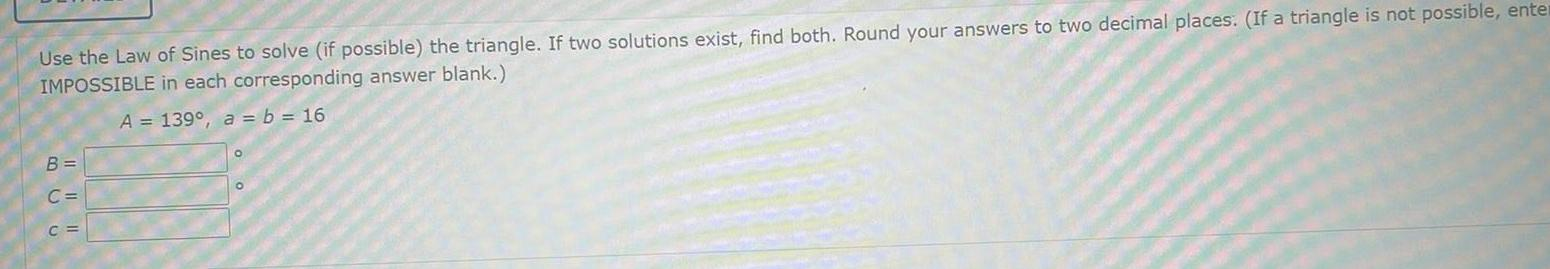Question:

# Use the Law of Sines to solve if possible the triangle If

Last updated: 9/20/2023Use the Law of Sines to solve if possible the triangle If two solutions exist find both Round your answers to two decimal places If a triangle is not possible enter IMPOSSIBLE in each corresponding answer blank A 139 a b 16 B C C